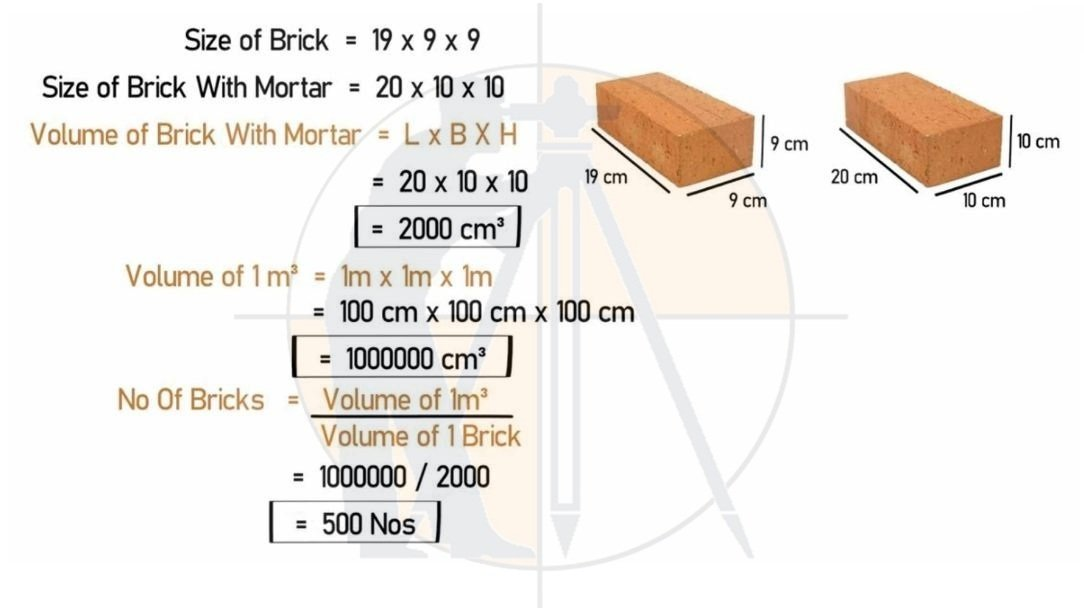Breaking News
Home / Civil Engineering / How To Calculate The Quantity Of Bricks in 1 m3

# How To Calculate The Quantity Of Bricks in 1 m3

## How To Calculate The Quantity Of Bricks in 1 m3

This question is mostly asked in interviews and commission exams. The right answer is 500 bricks are used in 1 m3. for this we know the standard size of a brick is 19″ x 9″ x 9″ And With mortar, it becomes 20′ x 10″ x 10′. So first we have to Find the Volume of Brick and then the volume of 1 m3 area. After we have the values of the volume of brick and volume of 1 m3 space, then we use a formula to find out how many no of brick are used in 1 m3.

The Formula is

No of Bricks = Volume of 1 m3 / Volume of 1 brick So the answer is 500 no of bricks are needed in 1 m3

Example: Size of Modular Bricks:

Length = 19cm

Height = 9cm

Therefore, Volume = 19x9x9 = 0.001539 m3

Number of Bricks in 1Cum without mortar = 1/0.001539

### How to calculate the number of bricks in 1 CFT wall.

= 649.772

= 650 nos.

Considering Mortar thickness =10mm

Size of Bricks with Mortar = 20cm x 10cm x 10cm

Volume of 1 Brick with Mortar =20x10x10 m3 (1)

Volume of 1Cum = 1x1x1m

= 100cm x 100cm x100cm (2)

Dividing equation (2) by (1)

Number of Bricks = (100X100X100) / (20X10X10)

= 500 Nos.### THANKS.